Next: Conclusions Up: ANGULAR-MOMENTUM PROJECTION OF CRANKED Previous: Angular-Momentum Projection

# Application to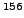Gd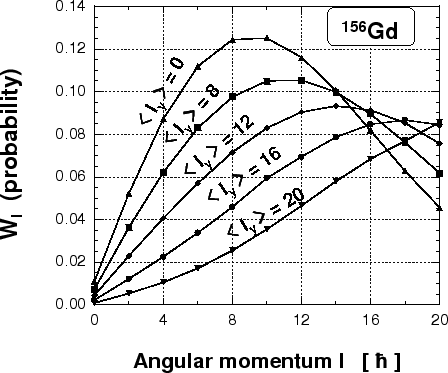We solve the cranked SHF equations forGd by using the code HFODD for the SIII Skyrme-force parameters and spherical harmonic-oscillator basis composed of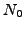=10 shells. Then, we calculate the Hamiltonian and overlap kernels by using 50 Gauss-Chebyshev integration points in the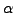and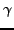directions and 50 Gauss-Legendre points in the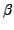direction. Figure 1 shows probability distributions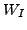of even angular-momentum components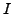in the intrinsic cranked-SHF states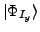constrained to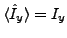. The probability of finding thecomponent can be calculated from: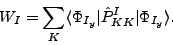(10)

The curves correspond to cranking wave functions with averaged angular momenta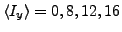, and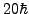. One can see that for low angular momenta (e.g., for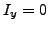) the maxima of the distributions do not lie near the same values of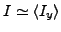. Similar results have been obtained by Islam et al. and Baye et al..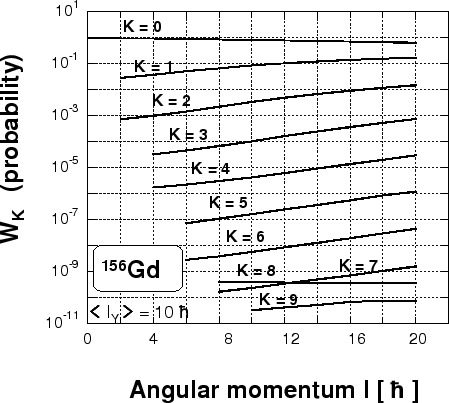In Fig. 2 we show similar probability distributions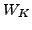of the projections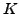,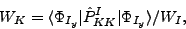(11)

projected from the state with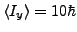inGd. One can see that the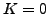component dominates for all angular momenta, while the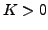components increase with the increasing angular momentum. The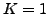component is the second in magnitude after, which illustrates the build-up of the Coriolis coupling in a rotating intrinsic state. Note that only even values ofcan be projected from the non-rotating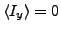state, while all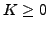appear in the cranked state.

In order to find energies of the AMP states, we solve Eq. (9) by diagonalizing first the norm matrix: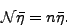(12)

The non-zero eigenvalues (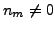) of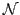are used afterwards to built the so-called natural states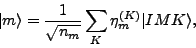(13)

that span the subspace called collective subspace. Final diagonalization of the HW equation (9) is performed within the collective subspace. In this subspace the problem reduces to the standard hermitian eigenvalue problem.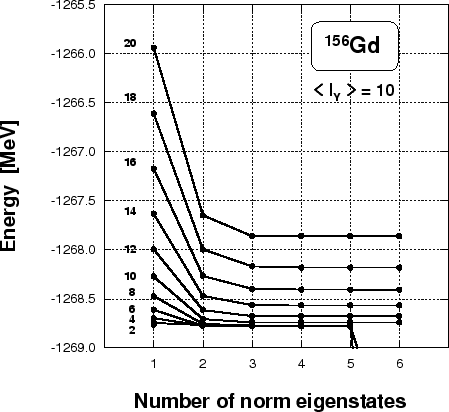In practical numerical applications we use the cut-off parameter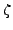to construct the collective subspace, by keeping only the norm eigenstates (12) with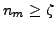. The test depicted in Fig. 3 shows the stability of projected solutions with respect to the number of the norm eigenstates kept in the collective subspace. The analysis was conducted for angular-momentum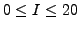states projected from the deformed solution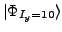obtained by solving the cranked SHF equations with the constraint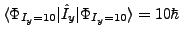. The test clearly shows that, starting from a certain point, the obtained solutions are stable (plateau condition). Only for very small values of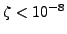the method becomes numerically unstable.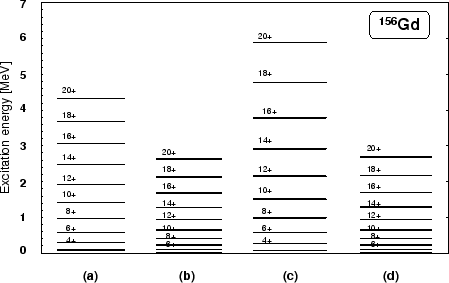Figure 4 shows rotational bands (b)-(d) calculated inGd in comparison with the experimental data (a). Band (b) represents the average mean-field energies obtained from the cranked SHF calculations by constraining solutions to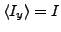. In bands (c) and (d), we show energies obtained by the AMP from theand, respectively. We see, that band (c) is much higher than bands (b) and (d), which indicates that the PAV from thestate is not an adequate method of describing nuclear rotation. Note that the mean-field energies (b) and the AMP energies (d) corresponding toturn out to be very similar to one another. This shows that the AMP from thestates constitutes a correct symmetry restoration of the approximate VAP solutions realized by the cranking procedure. The remaining discrepancy with experimental data must be attributed to pairing correlations, which are not included in our SHF solutions.

When calculating the Hamiltonian kernels within the LEDF approach, one has to use transition density matrices between rotated states,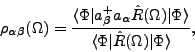(14)

which are singular whenever the rotated states are orthogonal. In particular, the transition multipole moments,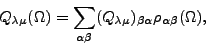(15)

become singular for certain values of the Euler angles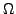. This is illustrated in Fig.  5, which shows absolute values of the neutron and proton overlap kernels in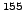Eu (left panel), and the transition quadrupole momentsinEu andGd (right panel). Calculations have been performed for axial shapes of nuclei, for which only the rotation about the Euler anglematters. The neutron overlap kernel corresponds to an even number of particles (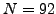), and is always positive, although at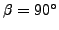it becomes as small as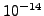. On the other hand, the proton overlap kernel corresponds to an odd number of particles (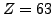), and it three times changes the sign in the interval of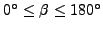. Consequently, the transition quadrupole moment ofGd is a regular function, while that ofEu has three poles.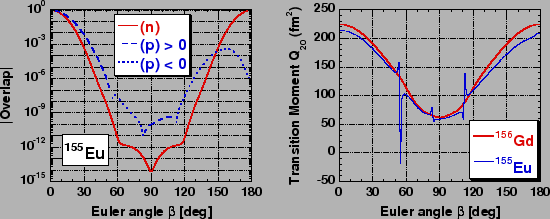Of course, when calculating the matrix elements of multipole operators between the rotated states, the transition matrix elements (15) are multiplied by the overlap kernels and the poles disappear. However, such a compensation is absent for kernels corresponding to higher powers of densities, viz. for the density-dependent terms of the Skyrme interactions, or for the direct-Coulomb-energy terms, or for the exchange-Coulomb-energy terms in the Slater approximation. How to treat such singular kernels within the AMP methods is currently an open and unsolved problem, similarly as is the case for the particle-number-projection methods recently discussed in Ref..Next: Conclusions Up: ANGULAR-MOMENTUM PROJECTION OF CRANKED Previous: Angular-Momentum Projection
Jacek Dobaczewski 2006-10-30